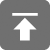# 工学1号馆

home

## Project Euler 23–Non-abundant sums

Wu Yudong    August 12, 2018     欧拉计划   550

A perfect number is a number for which the sum of its proper divisors is exactly equal to the number. For example, the sum of the proper divisors of 28 would be 1 + 2 + 4 + 7 + 14 = 28, which means that 28 is a perfect number.

A number n is called deficient if the sum of its proper divisors is less than n and it is called abundant if this sum exceeds n.

As 12 is the smallest abundant number, 1 + 2 + 3 + 4 + 6 = 16, the smallest number that can be written as the sum of two abundant numbers is 24. By mathematical analysis, it can be shown that all integers greater than 28123 can be written as the sum of two abundant numbers. However, this upper limit cannot be reduced any further by analysis even though it is known that the greatest number that cannot be expressed as the sum of two abundant numbers is less than this limit.

Find the sum of all the positive integers which cannot be written as the sum of two abundant numbers.

12是最小的过剩数，1 + 2 + 3 + 4 + 6 = 16。因此最小的能够写成两个过剩数之和的数字是24。经过分析，可以证明所有大于28123的数字都可以被写成两个过剩数之和。但是这个上界并不能被进一步缩小，即使我们知道最大的不能表示为两个过剩数之和的数字要比这个上界小。

#include <stdio.h>

int sum(int n)
{
int i;
int sum = 0;
int j = n / 2;
for (i = 1; i <= j; i++)
(n % i == 0) ? (sum += i) : (sum = sum);
return sum;
}
int main(void)
{
int b = { 0 }, c = {0}, x = 0;
for (int i = 2; i <= 28123; i++) {
x = sum(i);
if (x > i)
b[i] = 1;
}
for (int i = 2; i <= 28123; i++)
for (int j = 2; j <= 28123 - i; j++)
if (c[i + j] != 2)
c[i + j] = b[i] + b[j];
for (int i = 2; i <= 28123; i++)
if (c[i] != 2)
x += i;
printf("%d\n", x);
return 0;
}